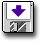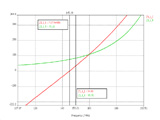Modeling a Center-Driven Dipole with CST

 Geometry and setup Geometry: L= 1 m, a = 0.5 mmModel parameters: Frequency: 50 MHz ~ 400 MHz Steady state accuracy limit: -60 dB Mesh definition: Mesh type: Hexahedral Mesh density control: Lines per wavelength = 30, Others = Default Special mesh properties: Refine at PEC/lossy metal edges by factor = 6, Others = Default Automesh Excitation: Discrete port (1 W, 50 ohms)cst_dipole.zip Simulation result Simulation time: 12 secs Number of mesh cells: 3600 Excitation duration: 2.031171e+001 ns Calculation time for excitation: 4 secs Number of calculated pulse widths: 3.49899 Simulated number of time steps: 8988 Maximum number of time steps: 51374 Decisions the user must make that affect the accuracy of the result Mesh density control: the minimum Lines per wavelength is 30. Special mesh properties: 6 or greater value is needed for 'Refine at PEC/lossy metal edges by factor' to obtain higher accuracy. Comments Adding the source requires a gap between the two wires. What is the source gap length?In this model, the interval between two antenna parts is 1 mm. What if we model the dipole as a flat ribbon?Substituting a 2-mm flat ribbon for a 0.5-mm radius round wire is not allowed in this model.
 Screen shotsFig. 4. Input impedance at the first resonant frequency